# Samacheer Kalvi 8th Maths Book Solutions Term 2 Chapter 2 | Algebra Answers For Tamil Nadu Board

Samacheer Kalvi 8th Maths Book Solutions Term 2 Chapter 2 – Algebra is available here. The Samacheer Kalvi 8th Maths book answers of Term 2 Chapter 2, available at BYJU’S, contain step by step explanations designed by our Mathematics experts. All these important questions are based on the new pattern prescribed by the Tamil Nadu board. Students can also get the solutions of other chapters on Samacheer Kalvi 8th Maths solutions. These Samacheer Kalvi Class 8 Maths Textbook Term 2 Chapter 2 questions can be solved by the students for extra practise and to get acquainted answering a wide range of questions of various difficulty level.

We have compiled here in this article below, the Samacheer Kalvi 8th Class Maths Textbook Term 2 Chapter 2 Questions with Solutions for the students to solve and practice.

## Samacheer Kalvi Class 8 Maths Book Term 2 Chapter 2 Questions and Solutions

Term 2 Chapter 2 of the Samacheer Kalvi 8th Maths guide will help the students to solve problems related to forming algebraic expressions, equations and its solutions, word problems that involve linear equations, graphing linear equations.

### Samacheer Kalvi 8th Maths Term 2 Chapter 2: Algebra Book Exercise 2.1 Questions and Solutions

Question 1: Find x: (3x – 2) / 4 – (x – 3) / 5 = -1

Solution:

(3x – 2) / 4 – (x – 3) / 5 = -1

Taking LCM of 4 and 5, 20 is obtained.

[3x * 5 – 2 * 5 – x * 4 – (-3 * 4)] / 20 = -1

[15x – 10 – 4x – (-12)] / 20 = -1

[15x – 10 – 4x + 12] / 20 = -1

[11x + 2] / 20 = -1

11x + 2 = -20

11x = -20 – 2

11x = -22

x = -2

Question 2: Solve for m: [m + 9] / [3m + 15] = 5 / 3

Solution:

[m + 9] / [3m + 15] = 5 / 3

3 (m + 9) = 5 (3m + 15)

3m + 27 = 15m + 75

3m – 15m = 75 – 27

-12m = 48

m = 48 / -12

m = -4

### Samacheer Kalvi 8th Maths Term 2 Chapter 2: Algebra Book Exercise 2.2 Questions and Solutions

Question 1: One number is seven times another. If their difference is 18, find the numbers.

Solution:

Let the numbers be x and y.

It is given that one number is seven times another and their difference is 18.

x = 7y —- (1)

x – y = 18 —- (2)

Substitute (1) in (2),

7y – y = 18

6y = 18

y = 18 / 6

y = 3

Substitute y = 3 in (1),

x = 7 * 3

x = 21

The numbers are 3 and 21.

Question 2: The sum of three consecutive odd numbers is 75. Which is the largest among them?

Solution:

The sum of three consecutive odd numbers is 75.

Odd numbers are 1, 3, 5, 7, ………

The difference between 2 consecutive odd numbers is always 2.

If the first odd number is x, then the second odd number would be x + 2, x + 4 and so on.

So,

x + x + 2 + x + 4 = 75

3x + 6 = 75

3x = 75 – 6

3x = 69

x = 69 / 3

x = 23

The odd numbers are 23, 23 + 2, 23 + 4.

So, they are 23, 25, 27.

The largest number is 27.

### Samacheer Kalvi 8th Maths Term 2 Chapter 2: Algebra Book Exercise 2.3 Questions and Solutions

Question 1: Find the quadrants without plotting the points on a graph sheet. (3, − 4), (5, 7), (2, 0), ( − 3, − 5), (4, − 3), ( − 7, 2), ( − 8, 0), (0, 10), ( − 9, 50).

Solution:

 (3, -4) (5, 7) (2, 0) (-3, -5) (4, -3) (-7, 2) (-8, 0) (0, 10) (-9, 50) IV I x-axis III IV II x-axis y-axis II

Question 2: Plot the following points in a graph sheet. A (5, 2), B (− 7, −3), C (−2, 4), D (−1, − 1), E (0, −5), F (2, 0), G (7, −4), H (−4, 0), I (2, 3), J (8, −4), K (0, 7).

Solution: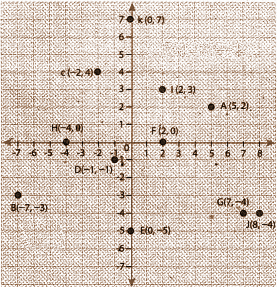### Samacheer Kalvi 8th Maths Term 2 Chapter 2: Algebra Book Exercise 2.4 Questions and Solutions

Question 1: If the points P(5, 3) Q(−3, 3) R(−3, −4) and S form a rectangle, then find the coordinate of S.

Solution:

On plotting the points on the graph,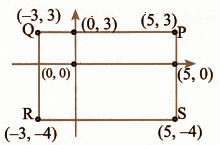Steps:

1] Plot points P, Q, R on the graph.

2] As it is a rectangle, RS will be parallel to the PQ and QR also parallel to PS.

3] S lies on the straight line from R parallel to the x-axis and straight line from P parallel to the y-axis.

4] Hence, S (5, -4).

Question 2: Draw the graph of the following equations

(i) x = −7

(ii) y = 6

Solution: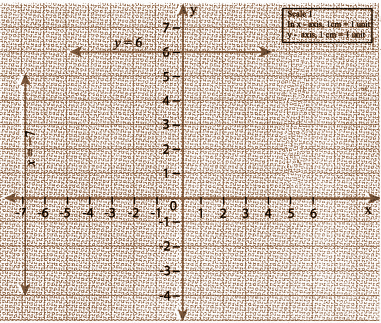### Samacheer Kalvi 8th Maths Term 2 Chapter 2: Algebra Book Exercise 2.5 Questions and Solutions

Question 1: Two equal sides of an isosceles triangle are 5y − 2 and 4y + 9 units. The third side is 2y + 5 units. Find ῾y᾿ and the perimeter of the triangle.

Solution:

Given that 5y – 2 and 4y + 9 are the equal sides of an isosceles triangle.

The 2 sides are equal.

5y – 2 = 4y + 9

5y – 4y = 9 + 2

y = 11

First side = 5y – 2 = 5 * 11 – 2 = 55 – 2 = 53

Second side = 4y + 9 = 4 * 11 + 9 = 44 + 9 = 53

Third side = 2y + 5 = 2 * 11 + 5 = 22 + 5 = 27

Perimeter is the sum of all 3 sides

P = 53 + 53 + 27 = 133

Question 2: Draw a graph for the following data: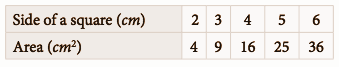Does the graph represent a linear relation?

Solution: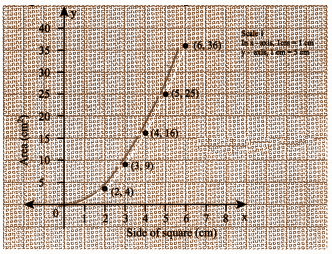It is not a linear relation.